This sounds like "given enough time, any number can be factorized i...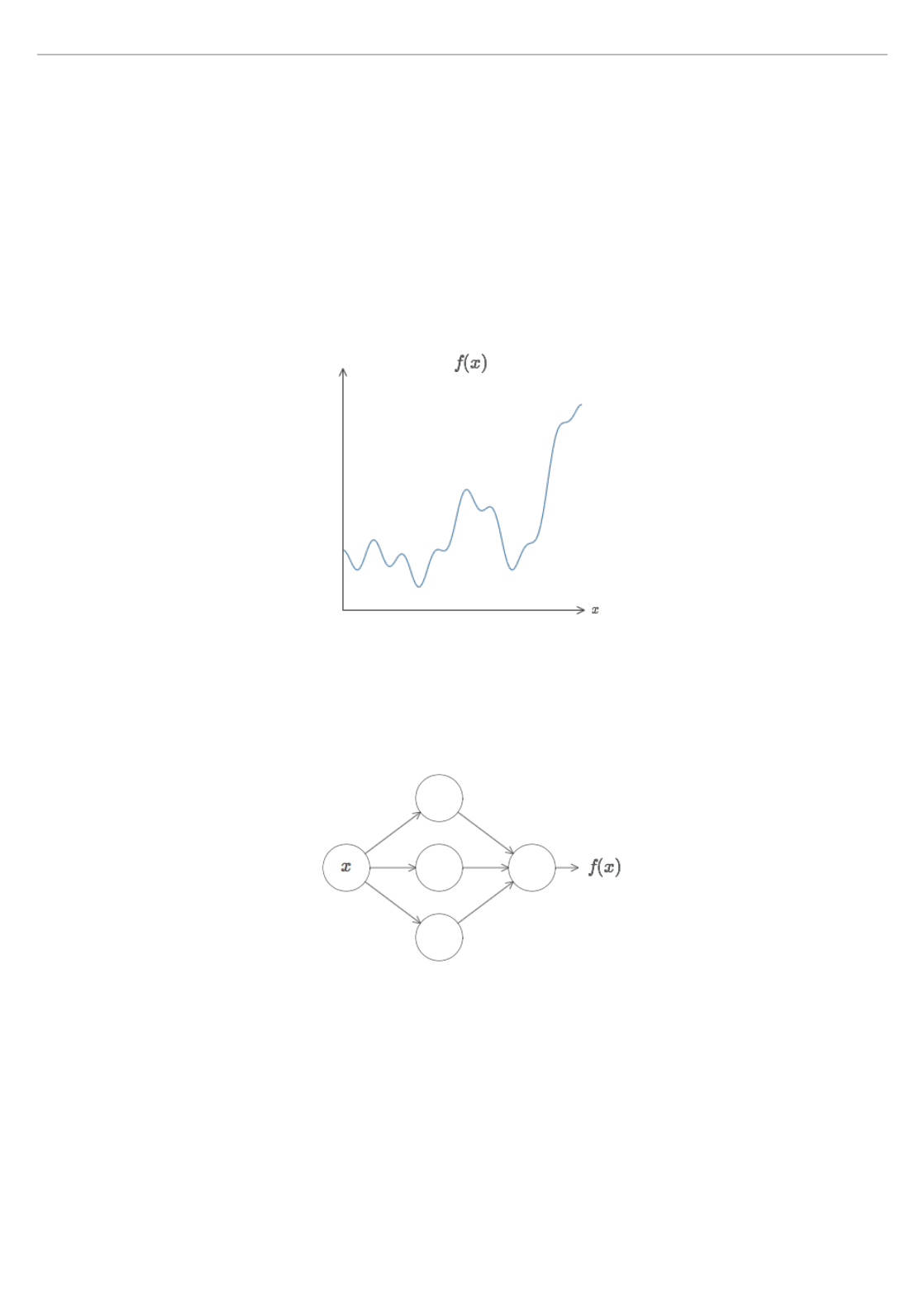One of the most striking facts about neural networks is that
they can compute any function at all. That is, suppose someone
hands you some complicated, wiggly function, :
No matter what the function, there is guaranteed to be a neural
network so that for every possible input, , the value (or
some close approximation) is output from the network, e.g.:
This result holds even if the function has many inputs,
, and many outputs. For instance, here's a
network computing a function with inputs and
outputs:
CHAPTER 4
A visual proof that neural nets can compute any function
f (x)
x
f (x)
f = f ( , , )
x
1
x
m
m = 3 n = 2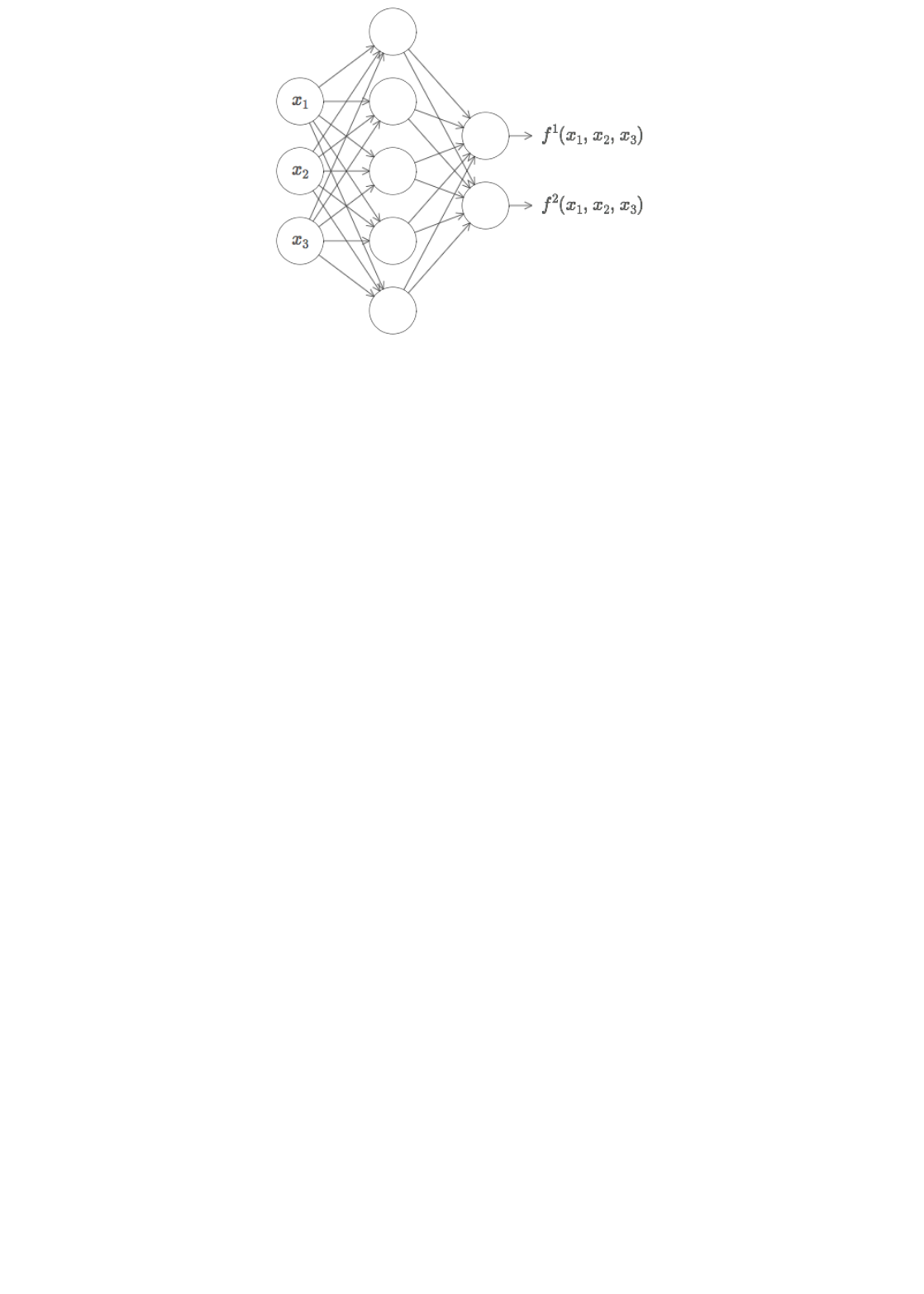This result tells us that neural networks have a kind of
universality. No matter what function we want to compute, we
know that there is a neural network which can do the job.
What's more, this universality theorem holds even if we restrict
our networks to have just a single layer intermediate between
the input and the output neurons - a so-called single hidden
layer. So even very simple network architectures can be
extremely powerful.
The universality theorem is well known by people who use
neural networks. But why it's true is not so widely understood.
Most of the explanations available are quite technical. For
instance, one of the original papers proving the result*
*Approximation by superpositions of a sigmoidal function, by George Cybenko
(1989). The result was very much in the air at the time, and several groups
proved closely related results. Cybenko's paper contains a useful discussion of
much of that work. Another important early paper is Multilayer feedforward
networks are universal approximators, by Kurt Hornik, Maxwell Stinchcombe,
and Halbert White (1989). This paper uses the Stone-Weierstrass theorem to
arrive at similar results.
did so using the Hahn-Banach theorem, the Riesz
Representation theorem, and some Fourier analysis. If you're a
mathematician the argument is not difficult to follow, but it's
not so easy for most people. That's a pity, since the underlying
reasons for universality are simple and beautiful.
In this chapter I give a simple and mostly visual explanation of
the universality theorem. We'll go step by step through the
underlying ideas. You'll understand why it's true that neural
networks can compute any function. You'll understand some of
the limitations of the result. And you'll understand how theresult relates to deep neural networks.
To follow the material in the chapter, you do not need to have
structured to be enjoyable as a self-contained essay. Provided
you have just a little basic familiarity with neural networks, you
should be able to follow the explanation. I will, however,
provide occasional links to earlier material, to help fill in any
Universality theorems are a commonplace in computer science,
so much so that we sometimes forget how astonishing they are.
But it's worth reminding ourselves: the ability to compute an
arbitrary function is truly remarkable. Almost any process you
can imagine can be thought of as function computation.
Consider the problem of naming a piece of music based on a
short sample of the piece. That can be thought of as computing
a function. Or consider the problem of translating a Chinese
text into English. Again, that can be thought of as computing a
function*
*Actually, computing one of many functions, since there are often many
acceptable translations of a given piece of text.
. Or consider the problem of taking an mp4 movie file and
generating a description of the plot of the movie, and a
discussion of the quality of the acting. Again, that can be
thought of as a kind of function computation*
*Ditto the remark about translation and there being many possible functions.
. Universality means that, in principle, neural networks can do
all these things and many more.
Of course, just because we know a neural network exists that
can (say) translate Chinese text into English, that doesn't mean
we have good techniques for constructing or even recognizing
such a network. This limitation applies also to traditional
universality theorems for models such as Boolean circuits. But,
as we've seen earlier in the book, neural networks have
powerful algorithms for learning functions. That combination
of learning algorithms + universality is an attractive mix. Up to
now, the book has focused on the learning algorithms. In this
chapter, we focus on universality, and what it means.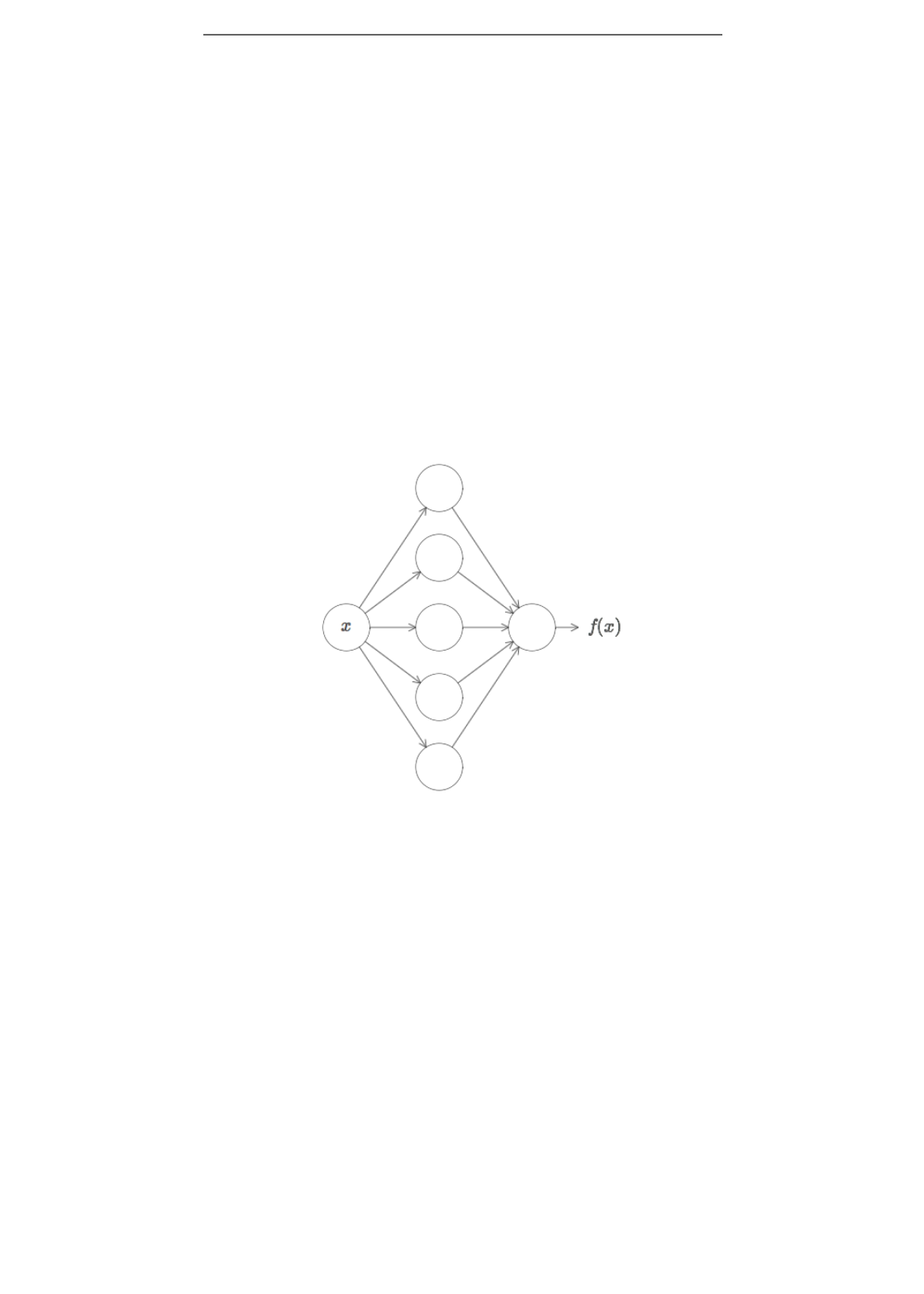Two caveats
Before explaining why the universality theorem is true, I want
to mention two caveats to the informal statement "a neural
network can compute any function".
First, this doesn't mean that a network can be used to exactly
compute any function. Rather, we can get an approximation
that is as good as we want. By increasing the number of hidden
neurons we can improve the approximation. For instance,
earlier I illustrated a network computing some function
using three hidden neurons. For most functions only a low-
quality approximation will be possible using three hidden
neurons. By increasing the number of hidden neurons (say, to
five) we can typically get a better approximation:
And we can do still better by further increasing the number of
hidden neurons.
To make this statement more precise, suppose we're given a
function which we'd like to compute to within some desired
accuracy . The guarantee is that by using enough hidden
neurons we can always find a neural network whose output
satisfies , for all inputs . In other words, the
approximation will be good to within the desired accuracy for
every possible input.
The second caveat is that the class of functions which can be
approximated in the way described are the continuous
functions. If a function is discontinuous, i.e., makes sudden,
sharp jumps, then it won't in general be possible to
f (x)
f (x)
ϵ > 0
g(x)
|g(x) f (x)| < ϵ
x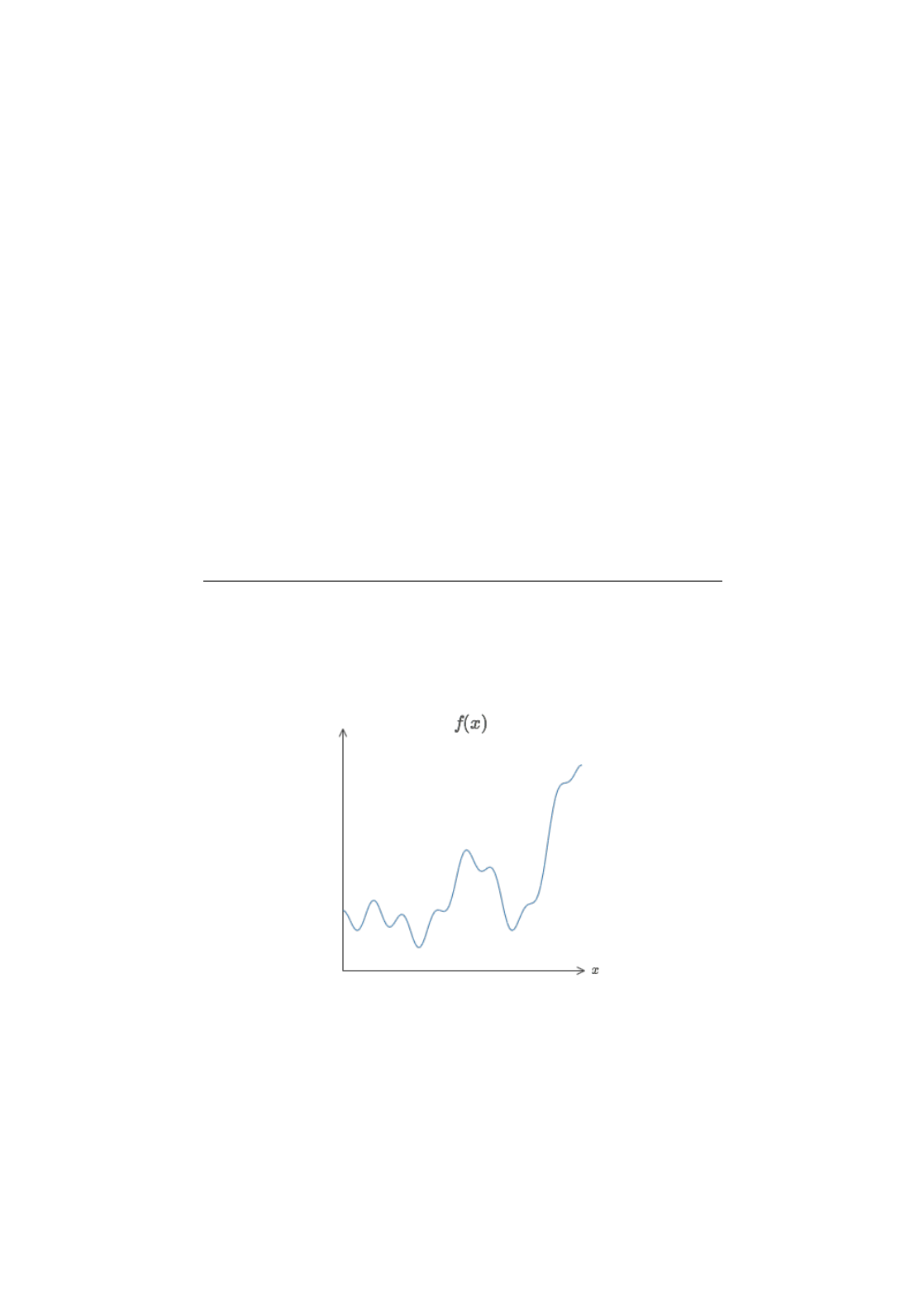approximate using a neural net. This is not surprising, since
our neural networks compute continuous functions of their
input. However, even if the function we'd really like to compute
is discontinuous, it's often the case that a continuous
approximation is good enough. If that's so, then we can use a
neural network. In practice, this is not usually an important
limitation.
Summing up, a more precise statement of the universality
theorem is that neural networks with a single hidden layer can
be used to approximate any continuous function to any desired
precision. In this chapter we'll actually prove a slightly weaker
version of this result, using two hidden layers instead of one. In
the problems I'll briefly outline how the explanation can, with a
few tweaks, be adapted to give a proof which uses only a single
hidden layer.
Universality with one input and one
output
To understand why the universality theorem is true, let's start
by understanding how to construct a neural network which
approximates a function with just one input and one output:
It turns out that this is the core of the problem of universality.
Once we've understood this special case it's actually pretty easy
to extend to functions with many inputs and many outputs.
To build insight into how to construct a network to compute ,
with two hidden neurons, and an output layer containing a
single output neuron:
f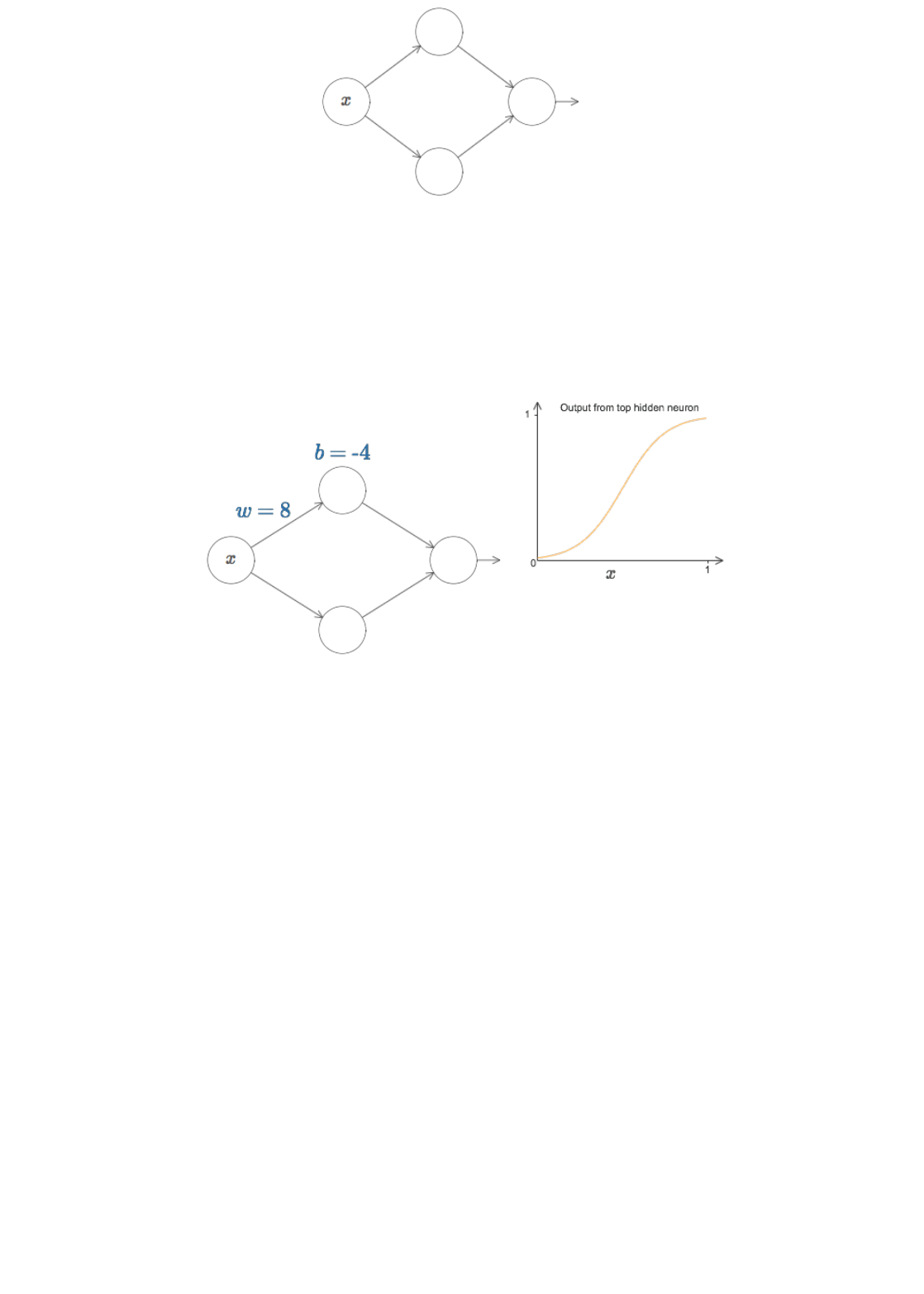To get a feel for how components in the network work, let's
focus on the top hidden neuron. In the diagram below, click on
the weight, , and drag the mouse a little ways to the right to
increase . You can immediately see how the function
computed by the top hidden neuron changes:
As we learnt earlier in the book, what's being computed by the
hidden neuron is , where is the
sigmoid function. Up to now, we've made frequent use of this
algebraic form. But for the proof of universality we will obtain
more insight by ignoring the algebra entirely, and instead
manipulating and observing the shape shown in the graph.
This won't just give us a better feel for what's going on, it will
also give us a proof*
*Strictly speaking, the visual approach I'm taking isn't what's traditionally
thought of as a proof. But I believe the visual approach gives more insight into
why the result is true than a traditional proof. And, of course, that kind of
insight is the real purpose behind a proof. Occasionally, there will be small
gaps in the reasoning I present: places where I make a visual argument that is
plausible, but not quite rigorous. If this bothers you, then consider it a
challenge to fill in the missing steps. But don't lose sight of the real purpose: to
understand why the universality theorem is true.
of universality that applies to activation functions other than
the sigmoid function.
To get started on this proof, try clicking on the bias, , in the
diagram above, and dragging to the right to increase it. You'll
w
w
σ(wx + b) σ(z) 1/(1 + )
e
z
b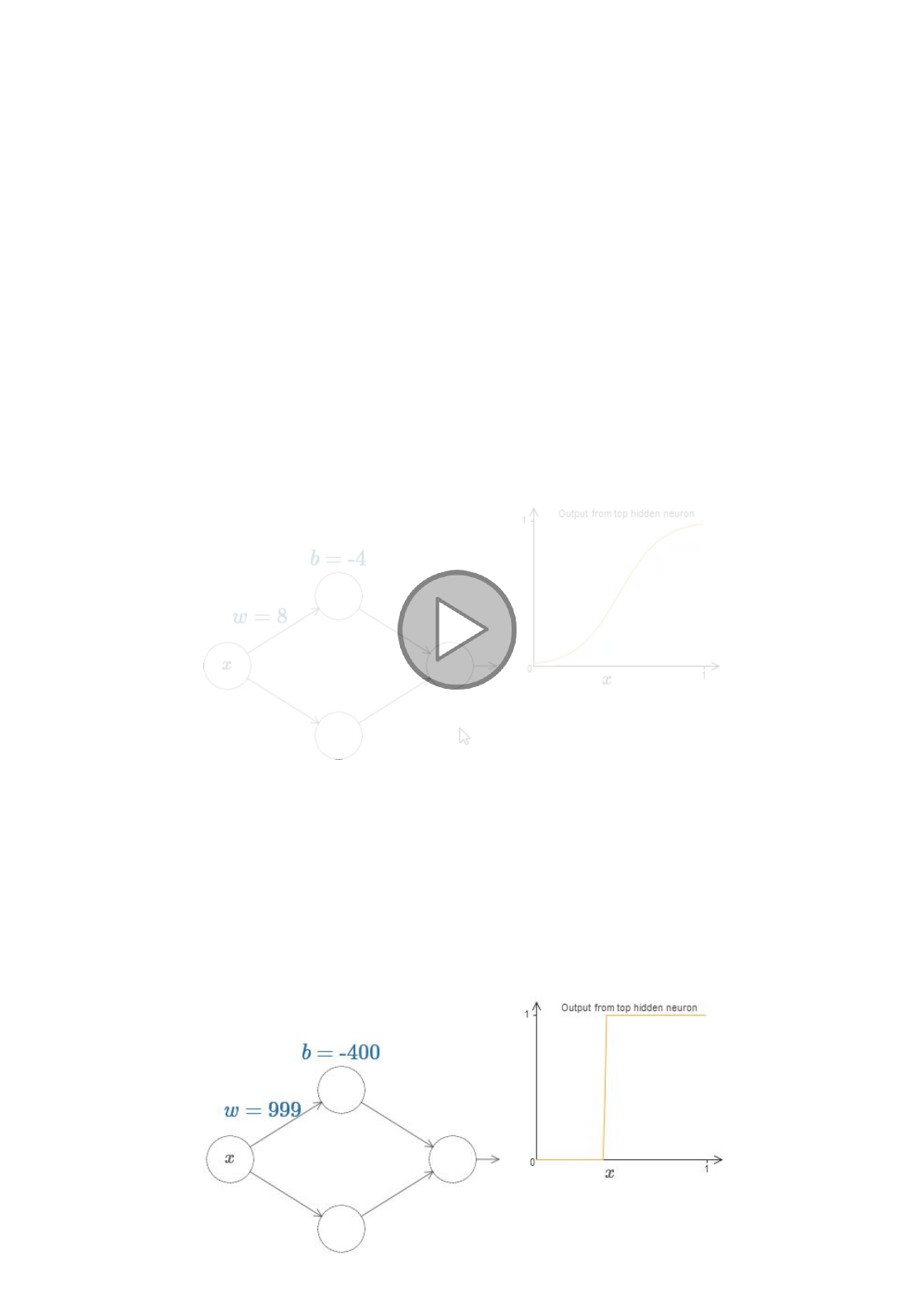see that as the bias increases the graph moves to the left, but its
shape doesn't change.
Next, click and drag to the left in order to decrease the bias.
You'll see that as the bias decreases the graph moves to the
right, but, again, its shape doesn't change.
Next, decrease the weight to around or . You'll see that as
you decrease the weight, the curve broadens out. You might
need to change the bias as well, in order to keep the curve in-
frame.
Finally, increase the weight up past . As you do, the
curve gets steeper, until eventually it begins to look like a step
function. Try to adjust the bias so the step occurs near .
The following short clip shows what your result should look
like. Click on the play button to play (or replay) the video:
We can simplify our analysis quite a bit by increasing the
weight so much that the output really is a step function, to a
very good approximation. Below I've plotted the output from
the top hidden neuron when the weight is . Note that
this plot is static, and you can't change parameters such as the
weight.
2 3
w = 100
x = 0.3
w = 999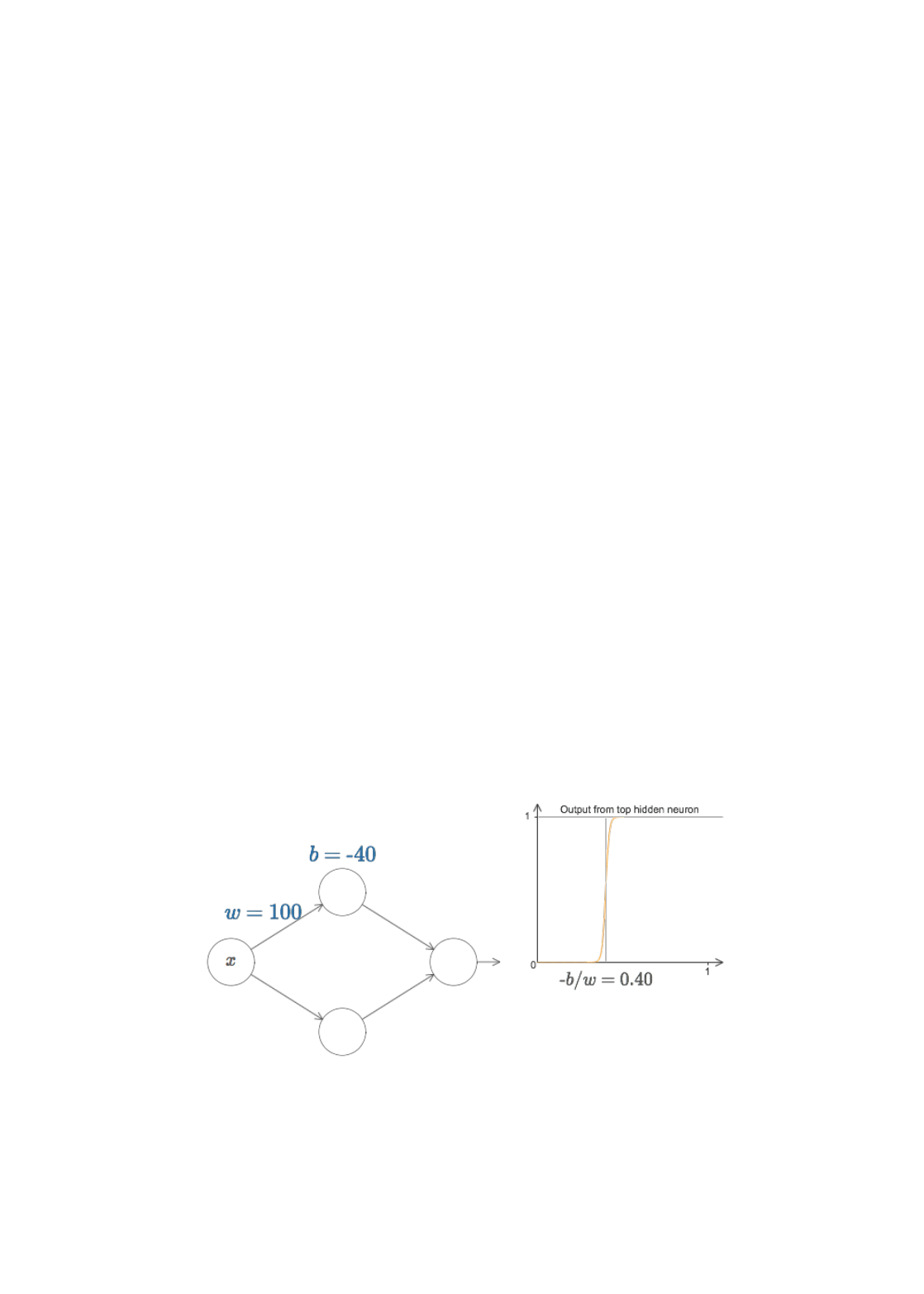It's actually quite a bit easier to work with step functions than
general sigmoid functions. The reason is that in the output
layer we add up contributions from all the hidden neurons. It's
easy to analyze the sum of a bunch of step functions, but rather
a bunch of sigmoid shaped curves. And so it makes things
much easier to assume that our hidden neurons are outputting
step functions. More concretely, we do this by fixing the weight
to be some very large value, and then setting the position of
the step by modifying the bias. Of course, treating the output as
a step function is an approximation, but it's a very good
approximation, and for now we'll treat it as exact. I'll come
back later to discuss the impact of deviations from this
approximation.
At what value of does the step occur? Put another way, how
does the position of the step depend upon the weight and bias?
To answer this question, try modifying the weight and bias in
the diagram above (you may need to scroll back a bit). Can you
figure out how the position of the step depends on and ?
With a little work you should be able to convince yourself that
the position of the step is proportional to , and inversely
proportional to .
In fact, the step is at position , as you can see by
modifying the weight and bias in the following diagram:
It will greatly simplify our lives to describe hidden neurons
using just a single parameter, , which is the step position,
. Try modifying in the following diagram, in order to
get used to the new parameterization:
w
x
w
b
b
w
s = b/w
s
s = b/w
s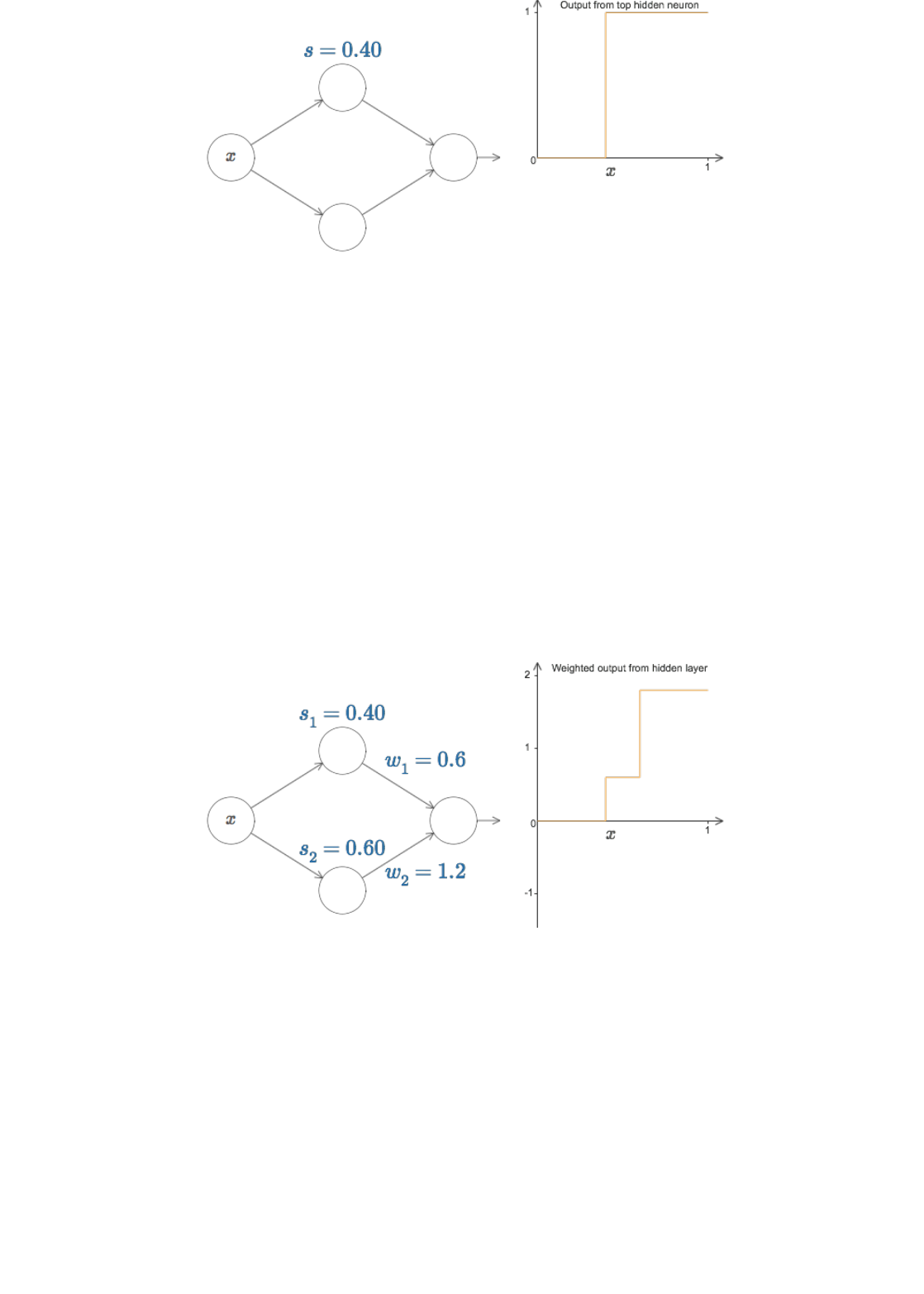As noted above, we've implicitly set the weight on the input
to be some large value - big enough that the step function is a
very good approximation. We can easily convert a neuron
parameterized in this way back into the conventional model, by
choosing the bias .
Up to now we've been focusing on the output from just the top
hidden neuron. Let's take a look at the behavior of the entire
network. In particular, we'll suppose the hidden neurons are
computing step functions parameterized by step points (top
neuron) and (bottom neuron). And they'll have respective
output weights and . Here's the network:
What's being plotted on the right is the weighted output
from the hidden layer. Here, and are the
outputs from the top and bottom hidden neurons, respectively*
*Note, by the way, that the output from the whole network is
, where is the bias on the output neuron. Obviously, this
isn't the same as the weighted output from the hidden layer, which is what
we're plotting here. We're going to focus on the weighted output from the
hidden layer right now, and only later will we think about how that relates to
the output from the whole network.
. These outputs are denoted with s because they're often
known as the neurons' activations.
w
b = ws
s
1
s
2
w
1
w
2
+
w
1
a
1
w
2
a
2
a
1
a
2
σ( + + b)
w
1
a
1
w
2
a
2
b
a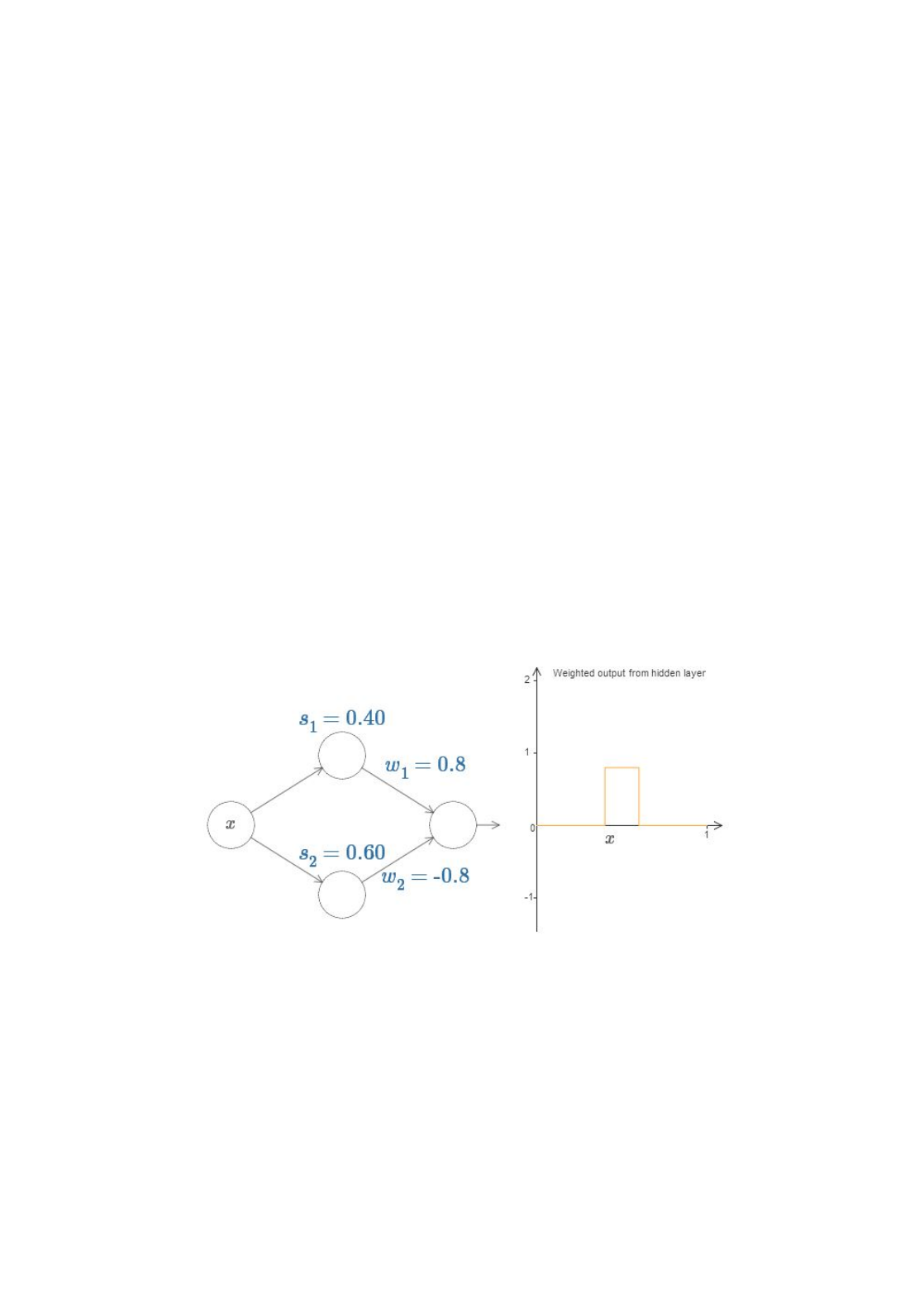Try increasing and decreasing the step point of the top
hidden neuron. Get a feel for how this changes the weighted
output from the hidden layer. It's particularly worth
understanding what happens when goes past . You'll see
that the graph changes shape when this happens, since we have
moved from a situation where the top hidden neuron is the first
to be activated to a situation where the bottom hidden neuron
is the first to be activated.
Similarly, try manipulating the step point of the bottom
hidden neuron, and get a feel for how this changes the
combined output from the hidden neurons.
Try increasing and decreasing each of the output weights.
Notice how this rescales the contribution from the respective
hidden neurons. What happens when one of the weights is
zero?
Finally, try setting to be and to be . You get a
"bump" function, which starts at point , ends at point , and
has height . For instance, the weighted output might look
like this:
Of course, we can rescale the bump to have any height at all.
Let's use a single parameter, , to denote the height. To reduce
clutter I'll also remove the " " and " " notations.
s
1
s
1
s
2
s
2
w
1
0.8
w
2
0.8
s
1
s
2
0.8
h
=
s
1
=
w
1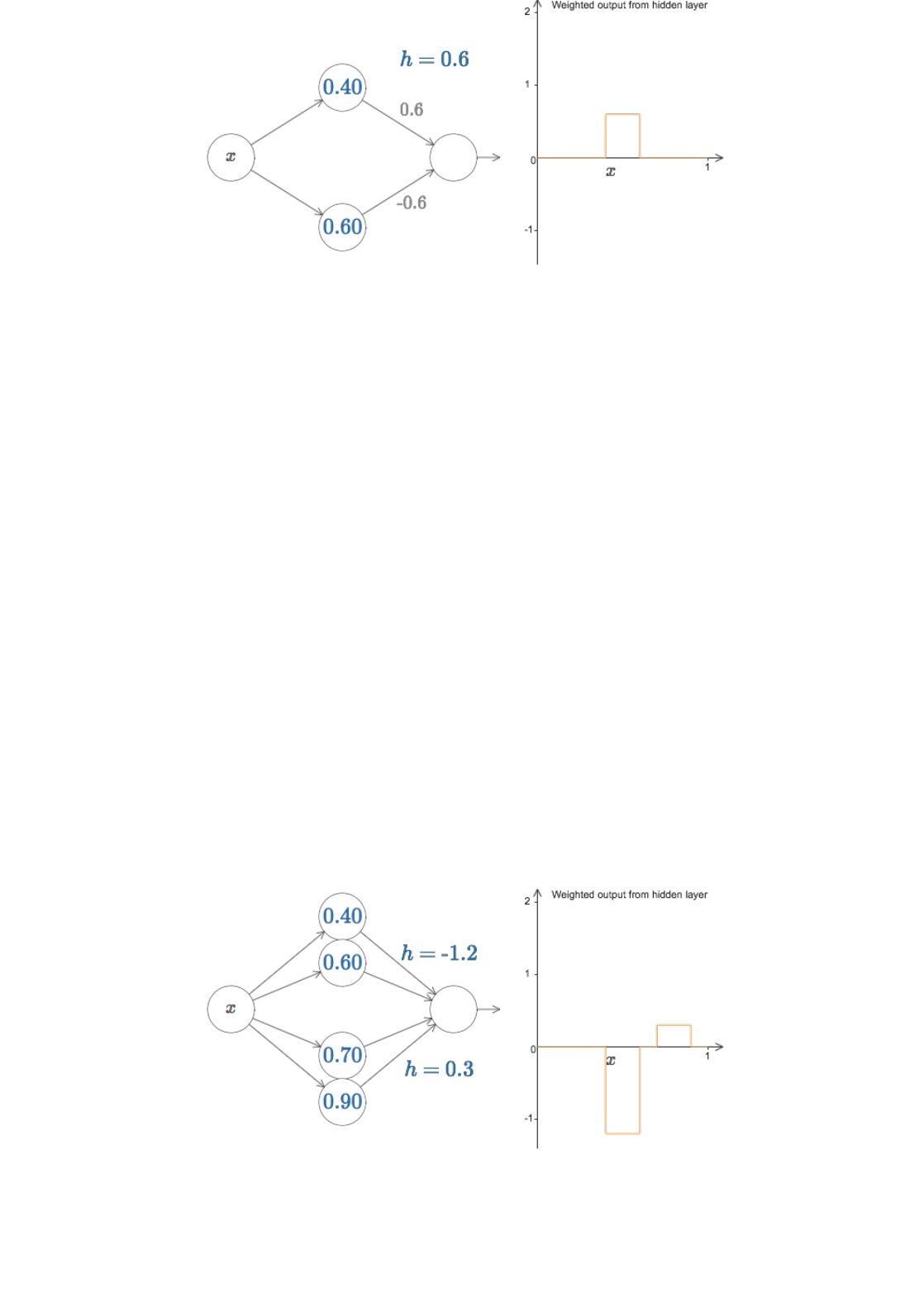Try changing the value of up and down, to see how the height
of the bump changes. Try changing the height so it's negative,
and observe what happens. And try changing the step points to
see how that changes the shape of the bump.
You'll notice, by the way, that we're using our neurons in a way
that can be thought of not just in graphical terms, but in more
conventional programming terms, as a kind of if-then-else
statement, e.g.:
if input >= step point:
add 1 to the weighted output
else:
add 0 to the weighted output
For the most part I'm going to stick with the graphical point of
view. But in what follows you may sometimes find it helpful to
switch points of view, and think about things in terms of if-
then-else.
We can use our bump-making trick to get two bumps, by gluing
two pairs of hidden neurons together into the same network:
I've suppressed the weights here, simply writing the values
for each pair of hidden neurons. Try increasing and decreasing
both values, and observe how it changes the graph. Move the
h
h
h